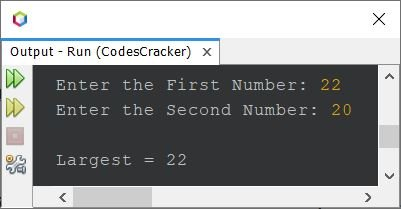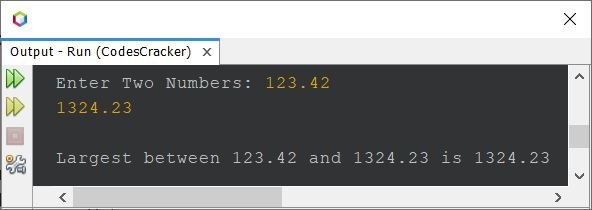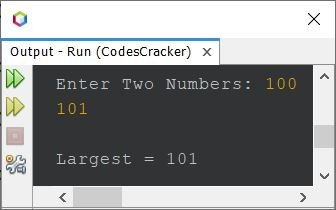# Java Program to Find Largest of Two Numbers

This article is created to cover some programs in Java that is used to find and print the largest or biggest between two given numbers. Here are the list of ways used to do the job:

• Find largest of two numbers using if...else
• Find largest of two numbers using conditional operator
• Find largest of two numbers using user-defined function

## Largest of Two Numbers using if-else

The question is, write a Java program to find largest between of two numbers. Both the number must be received by user at run-time of the program. The program given below is its answer:

```import java.util.Scanner;

public class CodesCracker
{
public static void main(String[] args)
{
int numberOne, numberTwo, largest;
Scanner scan = new Scanner(System.in);

System.out.print("Enter the First Number: ");
numberOne = scan.nextInt();
System.out.print("Enter the Second Number: ");
numberTwo = scan.nextInt();

if(numberOne>numberTwo)
largest = numberOne;
else
largest = numberTwo;

System.out.println("\nLargest = " +largest);
}
}```

The snapshot given below shows the sample run of above Java program, with user input 20 and 22 as two numbers:Here is another sample run with user input 22 and 20. That is, 22 as first and 20 as second number:But the problem with above program is, what if user enters same number, or a real number (containing decimal point). Therefore, I've modified the above program and created a new one to remove these two problems. Here is the program:

```import java.util.Scanner;

public class CodesCracker
{
public static void main(String[] args)
{
float a, b;
Scanner scan = new Scanner(System.in);

System.out.print("Enter Two Numbers: ");
a = scan.nextFloat();
b = scan.nextFloat();

if(a>b)
System.out.println("\nLargest between "+a+" and "+b+" is "+a);
else if(b>a)
System.out.println("\nLargest between "+a+" and "+b+" is "+b);
else
System.out.println("\nBoth Numbers are same.");

}
}```

Here is its sample run with user input 123.42 as first and 1324.23 as second number:## Largest of Two Numbers using Conditional Operator

With the use of conditional operator, the program becomes shorter. That is, the conditional operator helps in finding the largest of two numbers using single statement as shown in the program given below:

```import java.util.Scanner;

public class CodesCracker
{
public static void main(String[] args)
{
int a, b, big;
Scanner scan = new Scanner(System.in);

System.out.print("Enter Two Numbers: ");
a = scan.nextInt();
b = scan.nextInt();

big = (a>b) ? a : b;
System.out.println("\nLargest = " +big);
}
}```

Here is its sample run with user input 100 and 101 as two numbers:## Largest of Two Numbers using Function

This program uses a user-define function named LargestOfTwo(), that takes two numbers as its two arguments and returns the largest between its arguments.

```import java.util.Scanner;

public class CodesCracker
{
public static void main(String[] args)
{
Scanner scan = new Scanner(System.in);

System.out.print("Enter Two Numbers: ");
int x = scan.nextInt();
int y = scan.nextInt();

System.out.println("\nLargest = " +LargestOfTwo(x, y));
}
public static int LargestOfTwo(int a, int b)
{
return (a>b) ? a:b;
}
}```

This program produces same output as of previous program.

#### Same Program in Other Languages

Java Online Test

« Previous Program Next Program »